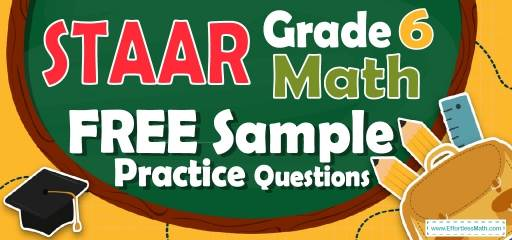# 6th Grade STAAR Math FREE Sample Practice QuestionsIf you are looking for sample practice questions to prepare your student for the 6th Grade STAAR Math test, you are in the right place. Our research team has collected a set of common questions in the 6th Grade STAAR Math test and provided it to you in this article. These questions focus on the important and common topics in the 6th Grade STAAR Math test exam. By avoiding unnecessary questions, we help the test takers to purposefully review the test resources and summarize them as best they can in the remaining time.

## 10 Sample 6th Grade STAAR Math Practice Questions

1- If the area of the following trapezoid is equal to A, which equation represents $$x$$?
$$\img {https://appmanager.effortlessmath.com/public/images/questions/131313444444444444444444444444444.JPG }$$

A. $$x = \frac{13}{A}$$

B. $$x = \frac{A}{13}$$

C. $$x=A+13$$

D. $$x=A-13$$

2- By what factor did the number below change from the first to the fourth number?
$$8, 104, 1352, 17576$$

A. 13

B. 96

C. 1456

D. 17568

3- 170 is equal to …

A. $$-20-(3×10)+(6×40)$$

B. $$((\frac{15}{8})×72 )+ (\frac{125}{5})$$

C. $$((\frac{30}{4} + \frac{15}{2})×8) – \frac{11}{2} + \frac{222}{4}$$

D. $$\frac{481}{6} + \frac{121}{3}+50$$

4- The distance between the two cities is 3,768 feet. What is the distance between the two cities in yards?

A. 1,256 yd

B. 11,304 yd

C. 45,216 yd

D. 3,768 yd

5- Mr. Jones saves $3,400 out of his monthly family income of$74,800. What fractional part of his income does Mr. Jones save?

A. $$\frac{1}{22}$$

B. $$\frac{1}{11}$$

C. $$\frac{3}{25}$$

D. $$\frac{2}{15}$$

6- What is the lowest common multiple of 12 and 20?

A. 60

B. 40

C. 20

D. 12

7- Based on the table below, which expression represents any value of f in terms of its corresponding value of $$x$$?
$$\img {https://appmanager.effortlessmath.com/public/images/questions/28282828288888888888888888888888888888888.JPG }$$

A. $$f=2x-\frac{3}{10}$$

B. $$f=x+\frac{3}{10}$$

C. $$f=2x+2 \frac{2}{5}$$

D. $$2x+\frac{3}{10}$$

8- Solve: 96 kg = … ?

A. 96 mg

B. 9,600 mg

C. 960,000 mg

D. 96,000,000 mg

9- Calculate the approximate area of the following circle? (the diameter is 25)
$$\img {https://appmanager.effortlessmath.com/public/images/questions/3030303000000000000000000000000000.JPG }$$

A. 78

B. 491

C. 157

D. 1963

10- The following graph shows the mark of six students in mathematics. What is the mean (average) of the marks?
$$\img {https://appmanager.effortlessmath.com/public/images/questions/313131313131111111111111111111111111111111111111.JPG }$$

A. 13

B. 13.5

C. 14

D. 1.5

## Best 6th Grade STAAR Math Exercise Resource for 2022

1- B
The area of the trapezoid is: area$$= \frac{(base \space 1+base \space 2)}{2}×$$height$$= (\frac{10 + 16}{2})x = A$$
$$→13x = A→x = \frac{A}{13}$$

2- A
$$\frac{104}{8}=13, \frac{1352}{104}=13, \frac{17576}{1352}=13$$
Therefore, the factor is 13

3- C
Simplify each option provided.
A. $$-20-(3×10)+(6×40)=-20-30+240=190$$
B. $$(\frac{15}{8})×72 + (\frac{125}{5}) =135+25=160$$
C. $$((\frac{30}{4} + \frac{15}{2})×8) – \frac{11}{2} + \frac{222}{4} = ((\frac{30 + 30}{4})×8)- \frac{11}{2}+ \frac{111}{2}=(\frac{60}{4})×8) + \frac{100}{2}= 120 + 50 = 170$$
D. $$\frac{481}{6} + \frac{121}{3}+50= \frac{481+242}{6}+50=120.5+50=170.5$$

4- A
1 yard $$= 3$$ feet
Therefore, $$3,768 \space ft × \frac{1 \space yd }{3 \space ft}=1,256 \space yd$$

5- A
3,400 out of 74,800 equals to $$\frac{3,400}{74,800}=\frac{17}{374}=\frac{1}{22}$$

6- A
Prime factorizing of $$20=2×2×5$$
Prime factorizing of $$12=2×2×3$$
LCM$$=2×2×3×5=60$$

7- C
Plugin the value of $$x$$ into the function f. First, plug-in 3.1 for$$x$$.
A. $$f=2x-\frac{3}{10}=2(3.1)-\frac{3}{10}=5.9≠8.6$$
B. $$f=x+\frac{3}{10}=3.1+\frac{3}{10}=3.4≠10.8$$
C. $$f=2x+2 \frac{2}{5}=2(3.1)+2 \frac{2}{5}=6.2+2.4=8.6$$
This is correct!
Plug in other values of $$x. (x=4.2)$$
$$f=2x+2\frac{2}{5} =2(4.2)+2.4=10.8$$
This one is also correct.
$$x=5.9$$
$$f=2x+2 \frac{2}{5}=2(5.9)+2.4=14.2$$
This one works too!
D. $$2x+\frac{3}{10}=2(3.1)+\frac{3}{10}=6.5≠8.6$$

8- D
1 kg$$= 1000$$ g and 1 g $$= 1000$$ mg
$$96$$ kg$$= 96 × 1000$$ g $$= 96 × 1000 × 1000 = 96,000,000$$ mg

9- B
The diameter of a circle is twice the radius. Radius of the circle is $$\frac{25}{2}$$.
Area of a circle = $$πr^2=π(\frac{25}{2})^2=156.25π=156.25×3.14=490.625≅491$$

10- B
Average (mean) $$=\frac{sum \space of \space terms}{number \space of \space terms}= \frac{10+11+15+14+15+17+12.5}{7}=13.5$$

The Most Comprehensive Review for 6th-Grade Students

### What people say about "6th Grade STAAR Math FREE Sample Practice Questions - Effortless Math: We Help Students Learn to LOVE Mathematics"?

No one replied yet.

X
41% OFF

Limited time only!

Save Over 41%

SAVE $12 It was$29.99 now it is \$17.99